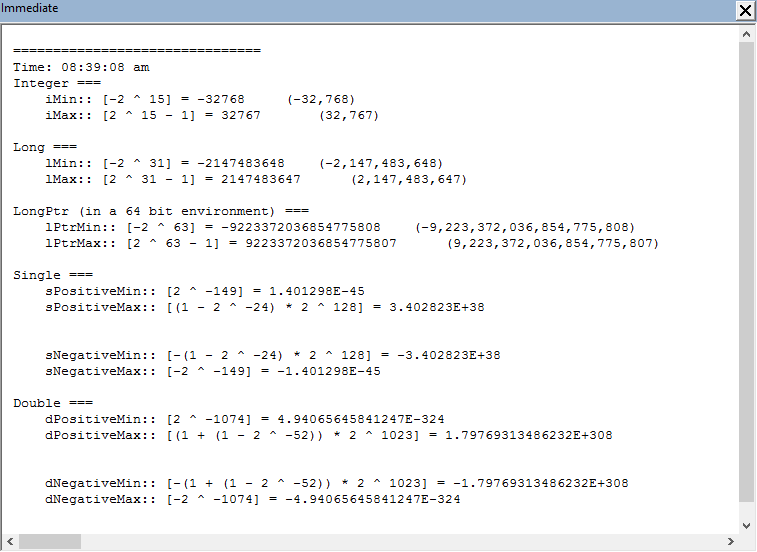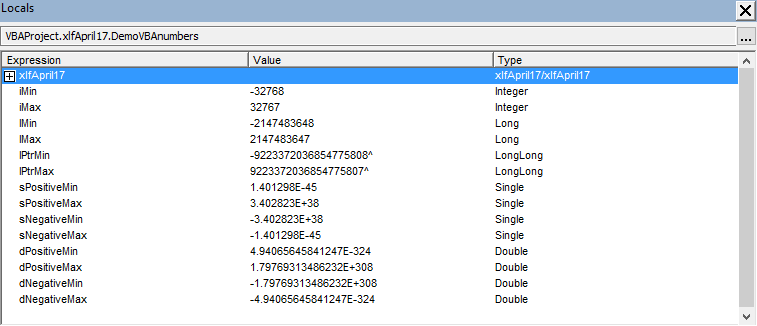# VBA number :: min to max

## VBA numbers - integer to floating-point

VBA and Excel specifications state the limits of numbers used in the application environment.

This module provides formulas written in VBA code, to return the minimum and maximum values (positive and negative) of:

• Integer
• Long
• LongPointer (LongPtr :: LongLong)
• Single precision floating point
• Double precision floating point

as specified in Excel VBA. The values for double precision floating point numbers are also applicable to formulas in Excel.

All formulas are binary (base 2) as depicted in code 1.

Code 1: VBA number :: minimum, maximum value demonstrator.

Sub DemoVBAnumbers()

Dim iMin As Integer
Dim iMax As Integer

Dim lMin As Long
Dim lMax As Long

Dim lPtrMin As LongPtr
Dim lPtrMax As LongPtr

Dim sPositiveMin As Single, sPositiveMax As Single
Dim sNegativeMin As Single, sNegativeMax As Single

Dim dPositiveMin As Double, dPositiveMax As Double
Dim dNegativeMin As Double, dNegativeMax As Double

Debug.Print vbNewLine & "==============================="
Debug.Print "Time: " & Format(Time, "hh:mm:ss am/pm")
' Integer (2 bytes)
Debug.Print "Integer ==="
iMin = -2 ^ 15
Debug.Print vbTab & "iMin:: [-2 ^ 15] = " & iMin & vbTab & "  (" & Format(iMin, "#,##0") & ")"
iMax = 2 ^ 15 - 1
Debug.Print vbTab & "iMax:: [2 ^ 15 - 1] = " & iMax & vbTab & vbTab & "  (" & Format(iMax, "#,##0") & ")"

' Long (4 bytes)
Debug.Print vbNewLine & "Long ==="
lMin = -2 ^ 31
Debug.Print vbTab & "lMin:: [-2 ^ 31] = " & lMin & vbTab & "  (" & Format(lMin, "#,##0") & ")"
lMax = 2 ^ 31 - 1
Debug.Print vbTab & "lMax:: [2 ^ 31 - 1] = " & lMax & vbTab & "  (" & Format(lMax, "#,##0") & ")"

' LongPtr
Debug.Print vbNewLine & "LongPtr (in a 64 bit environment) ==="
' Long integer on 32-bit systems
' LongLong integer on 64-bit systems
lPtrMin = -2 ^ 63
Debug.Print vbTab & "lPtrMin:: [-2 ^ 63] = " & lPtrMin & vbTab & "  (" & Format(lPtrMin, "#,##0") & ")"
lPtrMax = 2 ^ 63 - 1
Debug.Print vbTab & "lPtrMax:: [2 ^ 63 - 1] = " & lPtrMax & vbTab & "  (" & Format(lPtrMax, "#,##0") & ")"

' Single precision floating point (4 bytes, 32 bits)
Debug.Print vbNewLine & "Single ==="
sPositiveMin = 2 ^ -149
Debug.Print vbTab & "sPositiveMin:: [2 ^ -149] = " & sPositiveMin
sPositiveMax = (1 - 2 ^ -24) * 2 ^ 128
Debug.Print vbTab & "sPositiveMax:: [(1 - 2 ^ -24) * 2 ^ 128] = " & sPositiveMax

Debug.Print vbNewLine
sNegativeMin = -(1 - 2 ^ -24) * 2 ^ 128
Debug.Print vbTab & "sNegativeMin:: [-(1 - 2 ^ -24) * 2 ^ 128] = " & sNegativeMin
sNegativeMax = -2 ^ -149
Debug.Print vbTab & "sNegativeMax:: [-2 ^ -149] = " & sNegativeMax

' Double precision floating point (8 bytes, 64 bits)
Debug.Print vbNewLine & "Double ==="
dPositiveMin = 2 ^ -1074
Debug.Print vbTab & "dPositiveMin:: [2 ^ -1074] = " & dPositiveMin
dPositiveMax = (1 + (1 - 2 ^ -52)) * 2 ^ 1023
Debug.Print vbTab & "dPositiveMax:: [(1 + (1 - 2 ^ -52)) * 2 ^ 1023] = " & dPositiveMax

Debug.Print vbNewLine
dNegativeMin = -(1 + (1 - 2 ^ -52)) * 2 ^ 1023
Debug.Print vbTab & "dNegativeMin:: [-(1 + (1 - 2 ^ -52)) * 2 ^ 1023] = " & dNegativeMin
dNegativeMax = -2 ^ -1074
Debug.Print vbTab & "dNegativeMax:: [-2 ^ -1074] = " & dNegativeMax

Stop    ' halt code to view Locals Window

End Sub



The output from code 1 is sent to the Immediate Window, see figure 1, and the Locals Window in figure 2.Fig 1: Immediate Window showing the limit values for VBA number types: integer, long, longptr (longlong), single, and double

Single precision floating point numbers occupy 32 bits (4 bytes) of computer memory. The sign occupies 1 bit, the exponent 8 bits, and the fraction 23 bits. The fraction component has a maximum of 7 digits.

Double precision floating point numbers occupy 64 bits (8 bytes) of computer memory. The sign occupies 1 bit, the exponent 11 bits, and the fraction 52 bits. The fraction component has a maximum of 15 digits.Fig 2: Locals Window showing the limit values for VBA number types: integer, long, longptr, single, and double

All numbers in Excel, as distinct from VBA, are double precision floating point, and the Excel specifications state that the:

• Largest allowed positive number via formula (in Excel):= 1.7976931348623158e+308 (Note: displays as 1.79769313486232E+308)
• Largest allowed negative number via formula (in Excel):= -1.7976931348623158e+308 (Note: displays as -1.79769313486232E+308)
• See rows 18 and 19 of Sheet1 of the Excel Web App #1 in figure 3

### References

IEEE, IEEE 754: Standard for Binary Floating-Point Arithmetic Accessed: 26 April 2017

Microsoft - support, Excel specifications and limits Accessed: 26 April 2017

Microsoft - msdn, VBA data type summary Accessed: 26 April 2017

O'Connor I, (2011), VBA data types pdf [19KB]. Accessed 22 July 2017

• Development platform: Excel 2016 (Office 365 ProPlus).
• First published: 26 April 2017
• Revised: Saturday 25th of February 2023 - 10:12 AM, [Australian Eastern Time (AET)]